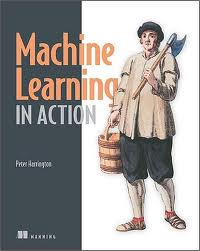Hi! We've renamed ScraperWiki.
The product is now QuickCode and the company is The Sensible Code Company.

# Book Review: Machine Learning in Action by Peter HarringtonMachine learning is about prediction, and prediction is a valuable commodity. This sounds pretty cool and definitely the sort of thing a data scientist should be into, so I picked up Machine Learning in Action by Peter Harrington to get an overview of the area.

Amongst the examples covered in this book are:

• Given that a customer bought these items, what other items are they likely to want?
• Is my horse likely to die from colic given these symptoms?
• Is this email spam?
• Given that these representatives have voted this way in the past, how will they vote in future?

In order to make a prediction, machine learning algorithms take a set of features and a target for a training set of examples. Once the algorithm has been trained, it can take new feature sets and make predictions based on them. Let’s take a concrete example: if we were classifying birds, the birds’ features would include the weight, size, colour and so forth and the target would be the species. We would train the algorithm on an initial set of birds where we knew the species, then we would measure the features of unknown birds and submit these to the algorithm for classification.

In this case, because we know the target – a species of bird – the algorithms we use would be referred to as “supervised learning.” This contrasts “unsupervised learning,” where the target is unknown and the algorithm is seeking to make its own classification. This would be equivalent to the algorithm creating species of birds by clustering those with similar features. Classification is the prediction of categories (i.e. eye colour, like/dislike), alternatively regression is used to predict the value of continuous variables (i.e. height, weight).

Machine learning in Action is divided into four sections that cover key elements and “additional tools” which includes algorithms for dimension reduction and MapReduce – a framework for parallelisation. Dimension reduction is the process of identifying which features (or combination of features) are essential to a problem.

Each section includes Python code that implements the algorithms under discussion and these are applied to some toy problems. This gives the book the air of Numerical Recipes in FORTRAN, which is where I cut my teeth on numerical analysis. The mixture of code and prose is excellent for understanding exactly how an algorithm works, but its better to use a library implementation in real life.

The algorithms covered are:

• Classification – k-Nearest Neighbours, decision trees, naive Bayes, logistic regression, support vector machines, and AdaBoost;
• Regression – linear regression, locally weighted linear regression, ridge regression, tree-based regression;
• Unsupervised learning – k-means clustering, apriori algorithm, FP-growth;
• Additional tools – principle component analysis and singular value decomposition.

Prerequisites for this book are relatively high: it assumes fair Python knowledge, some calculus, probability theory and matrix algebra.

I’ve seen a lot of mention of MapReduce without being clear what it was. Now I am more clear: it is a simple framework for carrying out parallel computation. Parallel computing has been around quite some time, the problem has always been designing algorithms that accommodate parallelisation (i.e. allow problems to be broken up into pieces which can be solved separately and then recombined). MapReduce doesn’t solve this problem but gives a recipe for what is required to run on commodity compute cluster.

As Harrington says: do you need to run MapReduce on a cluster to solve your data problem? Unless you are an operation on the scale of Google or Facebook then probably not. Current, commodity desktop hardware is surprisingly powerful particularly when coupled with subtle algorithms.

This book works better as an eBook than paper partly because the paper version is black and white and some figures require colour but the programming listings are often images and so the text remains small.